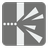Shooting Range
Elementary+
English RU

On a shooting range, shooters use a wall to practice, trying to hit it from various positions. Shooters do not have problems with vertical deviation, so we can use a simplified model for this mission. We placed a camera above the shooting range and use Cartesian coordinates to describe states. We know coordinates of wall (target) ends and shooting point. And we know the point where a bullet is after a small time. You should determine the result of the shot.

You are given coordinates for the end-points of the target wall on a grid. In addition, you have two points describing the shot: A starting point where the bullet was fired, and a second point indicating the location of the bullet after a specified length of time. You should calculate the result of the shot, specifically if a bullet hit the wall in the center, then it's 100 points. Awarded points reduce in relation to the distance from the center. An impact on the end of the wall is 0 points and a missed shot deducts a point (-1). The result should be rounded to the nearest integer.Input: Four arguments. Two wall ends, a firing point and a later point as tuples of two numbers.

Output: The results as an integer from -1 to 100.

Example:

```shot((2, 2), (5, 7), (11, 2), (8, 3)) == 100
shot((2, 2), (5, 7), (11, 2), (7, 2)) == 0
shot((2, 2), (5, 7), (11, 2), (8, 4)) == 29
shot((2, 2), (5, 7), (11, 2), (9, 5)) == -1
shot((2, 2), (5, 7), (11, 2), (10.5, 3)) == -1
```

How it is used: This concept can be used for modeling software and could help to determine the visibility of an object from a particular vantage point, or to calculate the trajectory of an object. For example, the trajectory of the ball in Pong, or a missile in Missile Command.

Preconditions:
all(all(0 < i < 100 for i in coor) for coor in args)
wall1, wall2 and shot_point are not collinear.